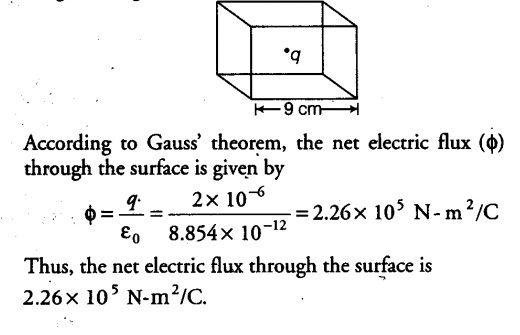# What is the net electric flux through the surface?

A point charge of 2.0 µC is at the centre of a cubic Gaussian surface 9.0 cm on edge. What is the net electric flux through the surface?

Let us consider a charge q is placed at the centre of a cubic Gaussian surface. As per the question, q = 2µC
= 2 x {{10}^{-6}} C
Length of edge, = 9cm